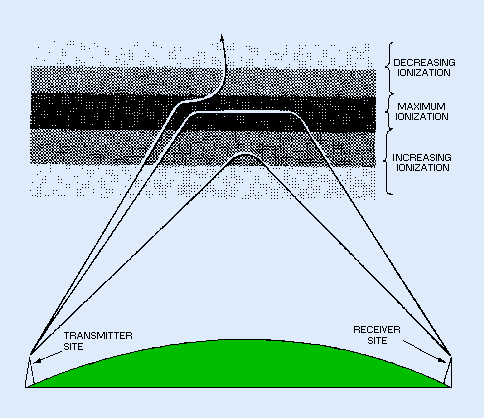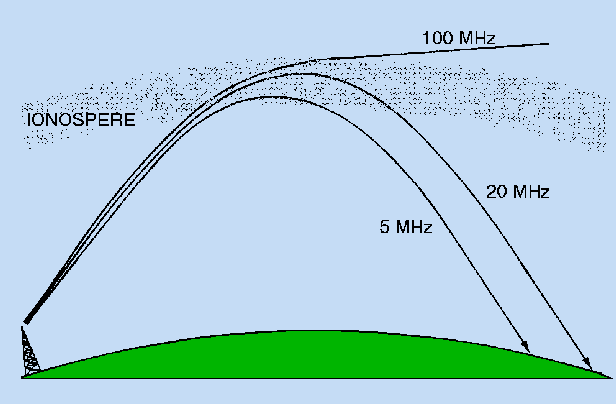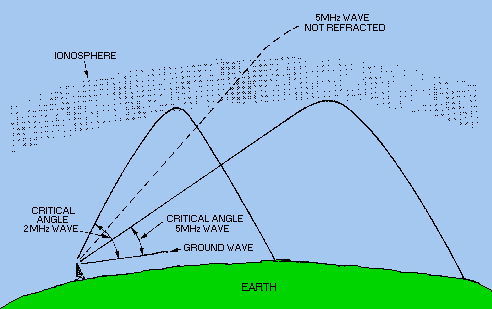Custom SearchWhen a radio wave is transmitted into an ionized layer, refraction, or bending of the wave, occurs. As we discussed earlier, refraction is caused by an abrupt change in the velocity of the upper part of a radio wave as it strikes or enters a new medium. The amount of refraction that occurs depends on three main factors: (1) the density of ionization of the layer, (2) the frequency of the radio wave, and (3) the angle at which the wave enters the layer. Density of Layer Figure 2-15 illustrates the relationship between radio waves and ionization density. Each ionized layer has a central region of relatively dense ionization, which tapers off in intensity both above and below the maximum region. As a radio wave enters a region of INCREASING ionization, the increase in velocity of the upper part of the wave causes it to be bent back TOWARD the Earth. While the wave is in the highly dense center portion of the layer, however, refraction occurs more slowly because the density of ionization is almost uniform. As the wave enters into the upper part of the layer of DECREASING ionization, the velocity of the upper part of the wave decreases, and the wave is bent AWAY from the Earth. Figure 2-15. - Effects of ionospheric density on radio waves.If a wave strikes a thin, very highly ionized layer, the wave may be bent back so rapidly that it will appear to have been reflected instead of refracted back to Earth. To reflect a radio wave, the highly ionized layer must be approximately no thicker than one wavelength of the radio wave. Since the ionized layers are often several miles thick, ionospheric reflection is more likely to occur at long wavelengths (low frequencies). Frequency For any given time, each ionospheric layer has a maximum frequency at which radio waves can be transmitted vertically and refracted back to Earth. This frequency is known as the CRITICAL FREQUENCY. It is a term that you will hear frequently in any discussion of radio wave propagation. Radio waves transmitted at frequencies higher than the critical frequency of a given layer will pass through the layer and be lost in space; but if these same waves enter an upper layer with a higher critical frequency, they will be refracted back to Earth. Radio waves of frequencies lower than the critical frequency will also be refracted back to Earth unless they are absorbed or have been refracted from a lower layer. The lower the frequency of a radio wave, the more rapidly the wave is refracted by a given degree of ionization. Figure 2-16 shows three separate waves of different frequencies entering an ionospheric layer at the same angle. Notice that the 5-megahertz wave is refracted quite sharply. The 20-megahertz wave is refracted less sharply and returned to Earth at a greater distance. The 100-megahertz wave is obviously greater than the critical frequency for that ionized layer and, therefore, is not refracted but is passed into space. Figure 2-16. - Frequency versus refraction and distance.The rate at which a wave of a given frequency is refracted by an ionized layer depends on the angle at which the wave enters the layer. Figure 2-17 shows three radio waves of the same frequency entering a layer at different angles. The angle at which wave A strikes the layer is too nearly vertical for the wave to be refracted to Earth. As the wave enters the layer, it is bent slightly but passes through the layer and is lost. When the wave is reduced to an angle that is less than vertical (wave B), it strikes the layer and is refracted back to Earth. The angle made by wave B is called the CRITICAL ANGLE for that particular frequency. Any wave that leaves the antenna at an angle greater than the critical angle will penetrate the ionospheric layer for that frequency and then be lost in space. Wave C strikes the ionosphere at the smallest angle at which the wave can be refracted and still return to Earth. At any smaller angle, the wave will be refracted but will not return to Earth. Figure 2-17. - Different incident angles of radio waves.As the frequency of the radio wave is increased, the critical angle must be reduced for refraction to occur. This is illustrated in figure 2-18. The 2-megahertz wave strikes the layer at the critical angle for that frequency and is refracted back to Earth. Although the 5-megahertz wave (broken line) strikes the ionosphere at a lesser angle, it nevertheless penetrates the layer and is lost. As the angle is lowered from the vertical, however, a critical angle for the 5-megahertz wave is reached, and the wave is then refracted to Earth. Figure 2-18. - Effects of frequency on the critical angle.Q.20 What factor determines whether a radio wave is reflected or refracted by the ionosphere?Q.21 There is a maximum frequency at which vertically transmitted radio waves can be refracted back to Earth. What is this maximum frequency called?Q.22 What three main factors determine the amount of refraction in the ionosphere?Integrated Publishing, Inc. - A (SDVOSB) Service Disabled Veteran Owned Small Business